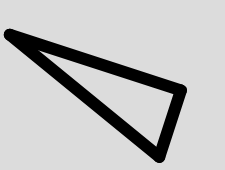# p5.js rotate() function

The rotate() function in p5.js is used to rotate a shape or the object using p5.js to a specified axis over a specified angle.

Syntax:

```rotate(angle, [axis])
```

Parameters: The function accepts single parameter as mentioned above and described below:

• angle: The angle of rotation which specified in radians or degrees.
• axis: the axis to rotate around

Below program illustrates the rotate() function in p5.js:
Example-1:

 `function` `setup() { ` `   `  `    ``// Create Canvas of given size ` `    ``createCanvas(380, 170); ` `} ` ` `  `function` `draw() { ` `   `  `    ``// Set the background color ` `    ``background(220); ` `   `  `    ``strokeWeight(12); ` `   `  `    ``//set strokeJoin function ` `    ``strokeJoin(ROUND); ` `   `  `    ``// rotation function ` `    ``rotate(PI / 10.0); ` `    ``line(20, 30, 200, 30); ` `    ``line(200, 30, 200, 100); ` `    ``line(200, 100, 20, 30); ` `} `

Output:Example-2:

 `function` `setup() { ` `   `  `    ``// Create Canvas of given size ` `    ``createCanvas(380, 170); ` `} ` ` `  `function` `draw() { ` `   `  `    ``// Set the background color ` `    ``background(220); ` `   `  `    ``strokeWeight(12); ` `   `  `    ``// rotation function ` `    ``rotate(PI / 7.0); ` `    ``textSize(30); ` `    ``text(``"GeeksForGeeks"``, 50, 50); ` `} `

Output:Reference: https://p5js.org/reference/#/p5/rotate

Whether you're preparing for your first job interview or aiming to upskill in this ever-evolving tech landscape, GeeksforGeeks Courses are your key to success. We provide top-quality content at affordable prices, all geared towards accelerating your growth in a time-bound manner. Join the millions we've already empowered, and we're here to do the same for you. Don't miss out - check it out now!